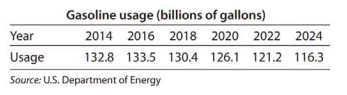# Modeling Gasoline usage In the United States, gasoline for motor vehicles is used continuously, and that usage, in billions of gallons per year, is shown in the table for selected years from 2014 and projected to 2024. Find a quadratic function that models these data, with t as the number of years past 2014. Report the model as G ( t ) with three significant digits. Evaluate ∫ 0 10 G ( t ) d t and tell what it represents.### Mathematical Applications for the ...

11th Edition
Ronald J. Harshbarger + 1 other
Publisher: Cengage Learning
ISBN: 9781305108042

#### Solutions

Chapter
Section### Mathematical Applications for the ...

11th Edition
Ronald J. Harshbarger + 1 other
Publisher: Cengage Learning
ISBN: 9781305108042
Chapter 13.2, Problem 69E
Textbook Problem
1 views

## Modeling Gasoline usage In the United States, gasoline for motor vehicles is used continuously, and that usage, in billions of gallons per year, is shown in the table for selected years from 2014 and projected to 2024. Find a quadratic function that models these data, with t as the number of years past 2014. Report the model as G ( t ) with three significant digits. Evaluate ∫ 0 10 G ( t ) d t and tell what it represents.This textbook solution is under construction.

### Still sussing out bartleby?

Check out a sample textbook solution.

See a sample solution

#### The Solution to Your Study Problems

Bartleby provides explanations to thousands of textbook problems written by our experts, many with advanced degrees!

Get Started